Open in App
Not now

# DFA that recognizes number of 0 is multiple of 3 on input {0,1}

• Difficulty Level : Medium
• Last Updated : 30 Jan, 2023

Finite Automata is known as a finite state machine that are acceptable otherwise not acceptable. on the input alphabet ‘0’ and 1′.

• Determine the initial state.
• The transition occurs on every input alphabet.
• Determine whether the self-loop should apply or not.
• Mark’s final state.

Designing DFA step by step :
Step-1:
Make initial state “A” then it is the possibility that there would not be any ‘0’ but have only ‘1’ in the string which is acceptable because 0 is divisible by 3.So, in this case, any number of 1’s can be present here and for this put self-loop of ‘1’ on initial state “A”.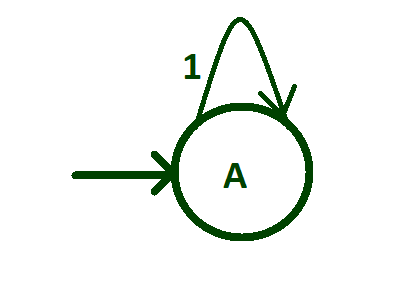Step-2:
Create transition of input alphabet ‘0’ from state “A” to state “B”.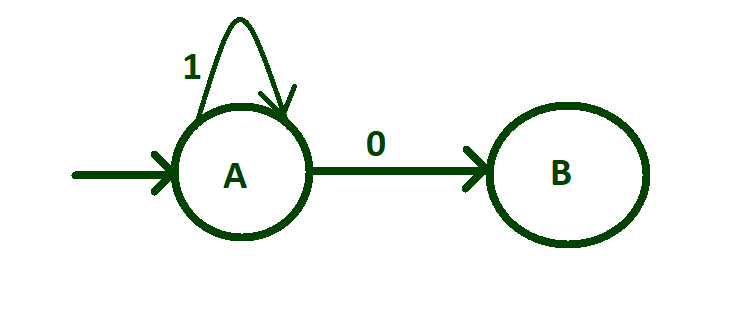Step-3:
After one ‘0’ any number of 1’s can be present i.e, no ‘1’ or more than one ‘1’.For this put self loop of ‘1’ on state “B”.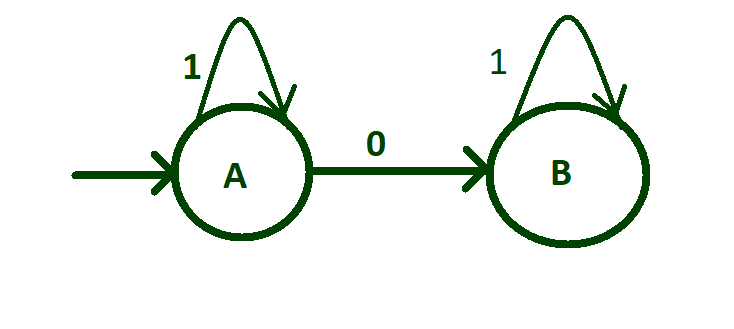Step-4:
Now create transition of input alphabet ‘0’ from state “B” to state “C” and after two 0’s any number of 1’s can be found in the string and for this put self loop of ‘1’ on initial state “C”.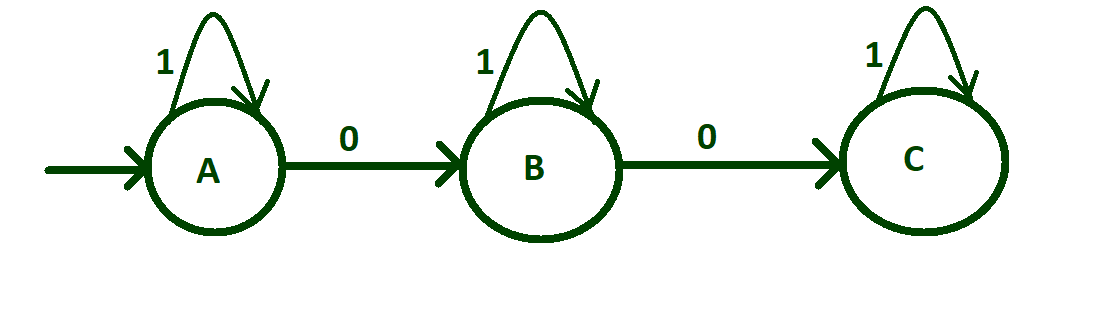Step-5:
Before transition of third ‘0’ we need to think about the logic so that after this transition the machine will accept string that have number of zeros divisible by 3.For this transit ‘o’ from state “C” to state “A”.AS third zero is reaching to state “A” so make state “A” be final state.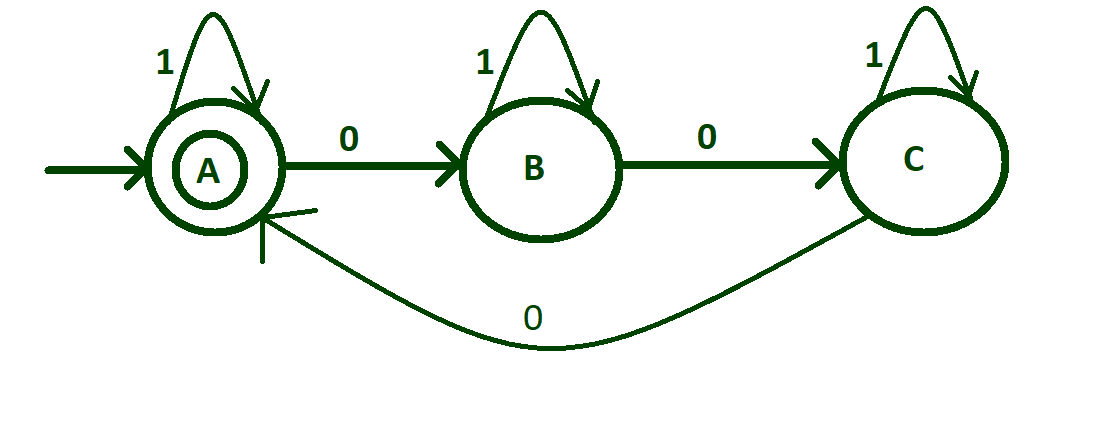Transition table of above DFA:

In above table, —> represents initial state and * represents final state. In this article, initial and final state is same which is final state.
Transition rules of above DFA: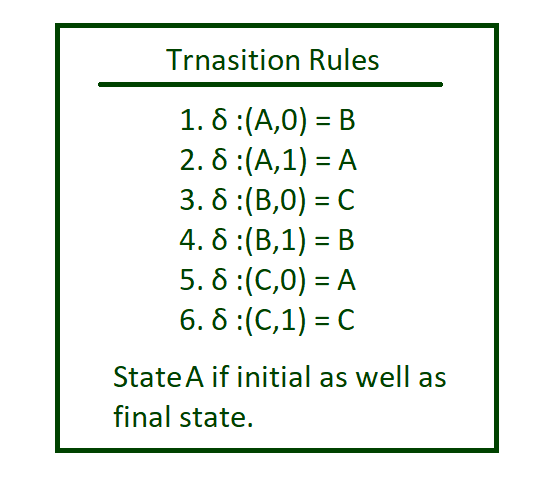Implementing :

## Java

 `// Java code for the above DFA``import` `java.util.*;` `class` `GFG{``     ` `// Function for the state A``static` `void` `checkStateA(String n)``{``    ` `    ``// Check length of n``    ``// is 0 then print``    ``// String accepted``    ``if` `(n.length() == ``0``)``        ``System.out.print(``"String accepted"``);``    ` `    ``// If 1 is found call function``    ``// checkStateA otherwise if 0``    ``// is found call function stateB``    ``else``    ``{``        ``if` `(n.charAt(``0``) == ``'1'``)``            ``checkStateA(n.substring(``1``));``        ``else``            ``stateB(n.substring(``1``));``    ``}``}``  ` `// Function for the state B``static` `void` `stateB(String n)``{``    ` `    ``// Check length of n``    ``// is 0 then print``    ``// String not accepted``    ``if` `(n.length() == ``0``)``        ``System.out.print(``"String not accepted"``);``    ` `    ``// If 1 is found call function``    ``// stateB otherwise if 0``    ``// is found call function stateC   ``    ``else``    ``{``        ``if` `(n.charAt(``0``) == ``'1'``)``            ``stateB(n.substring(``1``));``        ``else``            ``stateC(n.substring(``1``));``    ``}``}`` ` `// Function for the state C``static` `void` `stateC(String n)``{``    ` `    ``// Check length of n``    ``// is 0 then print``    ``// String not accepted``    ``if` `(n.length() == ``0``)``        ``System.out.print(``"String not accepted"``);``    ` `    ``// If 1 is found call function``    ``// stateC otherwise if 0``    ``// is found call function checkStateA``    ``else``    ``{``        ``if` `(n.charAt(``0``) == ``'1'``)``            ``stateC(n.substring(``1``));``        ``else``            ``checkStateA(n.substring(``1``));``    ``}``}`` ` `// Driver code``public` `static` `void` `main(String []args)``{``    ``Scanner sc = ``new` `Scanner(System.in);``    ` `    ``// Take String input``    ``String n = sc.nextLine();``    ` `    ``// Call checkStateA to``    ``// check the inputted String``    ``checkStateA(n);``}``}` `// This code is contributed by pratham76`

## Python3

 `# Python3 code for the above DFA``def` `checkStateA(n):``    ` `    ``# check length of n``    ``# is 0 then print``    ``# string accepted``    ``if``(``len``(n)``=``=` `0``):``        ``print``(``"string accepted"``)``        ` `    ``# if 1 is found call function``    ``# checkStateA otherwise if 0``    ``# is found call function stateB``    ``else``:   ``        ``if``(n[``0``]``=``=``'1'``):``            ``checkStateA(n[``1``:])``        ``else``:``            ``stateB(n[``1``:])`` ` ` ` `def` `stateB(n):``    ` `    ``# check length of n``    ``# is 0 then print``    ``# string not accepted``    ``if``(``len``(n)``=``=` `0``):``        ``print``(``"string not accepted"``)``        ` `    ``# if 1 is found call function``    ``# stateB otherwise if 0``    ``# is found call function stateC   ``    ``else``:   ``        ``if``(n[``0``]``=``=``'1'``):``            ``stateB(n[``1``:])``        ``else``:``            ``stateC(n[``1``:])``       ` `        ` `def` `stateC(n):``    ` `    ``# check length of n``    ``# is 0 then print``    ``# string not accepted``    ``if``(``len``(n)``=``=` `0``):``        ``print``(``"string not accepted"``)``        ` `    ``# if 1 is found call function``    ``# stateC otherwise if 0``    ``# is found call function checkStateA``    ``else``:   ``        ``if``(n[``0``]``=``=``'1'``):``            ``stateC(n[``1``:])``        ``else``:``            ``checkStateA(n[``1``:])``        ` `# take string input``n ``=` `input``()` `# call checkStateA``# to check the inputted string``checkStateA(n)`

## C#

 `// C# code for the above DFA``using` `System;``using` `System.Collections;``using` `System.Collections.Generic;``class` `GFG{``    ` `// Function for the state A``static` `void` `checkStateA(``string` `n)``{``  ``// check length of n``  ``// is 0 then print``  ``// string accepted``  ``if``(n.Length == 0)``    ``Console.Write(``"string accepted"``);` `  ``// if 1 is found call function``  ``// checkStateA otherwise if 0``  ``// is found call function stateB``  ``else``  ``{``    ``if``(n == ``'1'``)``      ``checkStateA(n.Substring(1));``    ``else``      ``stateB(n.Substring(1));``  ``}``}`` ` `// Function for the state B``static` `void` `stateB(``string` `n)``{``  ``// check length of n``  ``// is 0 then print``  ``// string not accepted``  ``if``(n.Length == 0)``    ``Console.Write(``"string not accepted"``);` `  ``// if 1 is found call function``  ``// stateB otherwise if 0``  ``// is found call function stateC   ``  ``else``{``    ``if``(n == ``'1'``)``      ``stateB(n.Substring(1));``    ``else``      ``stateC(n.Substring(1));``  ``}``}` `// Function for the state C``static` `void` `stateC(``string` `n)``{``  ``// check length of n``  ``// is 0 then print``  ``// string not accepted``  ``if``(n.Length == 0)``    ``Console.Write(``"string not accepted"``);` `  ``// if 1 is found call function``  ``// stateC otherwise if 0``  ``// is found call function checkStateA``  ``else``  ``{``    ``if``(n == ``'1'``)``      ``stateC(n.Substring(1));``    ``else``      ``checkStateA(n.Substring(1));``  ``}``}` `// Driver code``public` `static` `void` `Main(``string` `[]args)``{``  ``// take string input``  ``string` `n = Console.ReadLine();` `  ``// call checkStateA``  ``// to check the inputted string``  ``checkStateA(n);``}``}` `// This code is contributed by rutvik_56`

Time complexity: O(n) where n given string input

Auxiliary space: O(1)

My Personal Notes arrow_drop_up